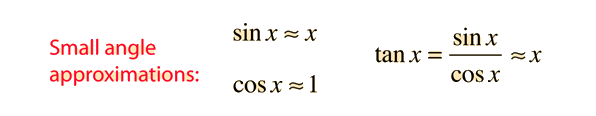# Paraxial Rays

The commonly used optical expressions like the lens equation are approximations which are only valid for light rays close to the optic axis for which the approximation sinθ ≈ θ is valid. Such rays are called "paraxial rays". The validity of this approximation can be evaluated by looking at the series representation of the trigonometric functions, and this small angle approximation is used in numerous applications.This approximation sin x ≈ x reaches a 1% error at about 14 degrees.

For applications involving refraction, Snell's law is expressed in terms of the trigonometric functions: n1sinθ1 = n2sinθ2. But for small angles, Snell's law can be approximated by n1θ1 ≈ n2θ2. The approximation tanθ ≈ θ is used in developing the expression for surface power, which in turn is used in the lens-maker's equation and from that the derivation of the thin lens equation, so the paraxial approximation is used in most common geometrical optics expressions.

Index

Lens concepts

References

Jenkins & White, 4th Ed Sec 2.12
Pedrotti & Pedrotti, Sec 3-5

 HyperPhysics***** Light and Vision R Nave
Go Back